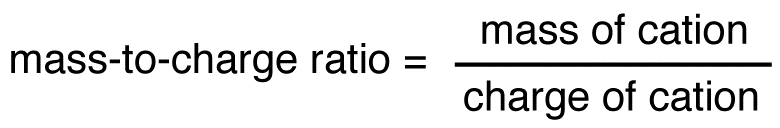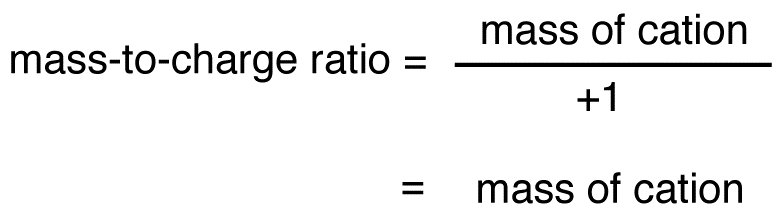# Mass-to-Charge Ratio

•• Contributed by Gamini Gunawardena
• Associate Professor (Chemistry) at Utah Valley University

In mass spectroscopy, the mass-to-charge ratio (symbols: m/z, m/e) of a cation is equal to the mass of the cation divided by its charge.Since the charge of cation formed in the mass spectrometer is almost always +1, the mass-to-charge ratio of a cation is usually equal to the mass of the cation.The mass of the molecular ion is equal to the molecular weight of the compound. Thus, the mass-to-charge ratio of the molecular ion is equal to the molecular weight of the compound.